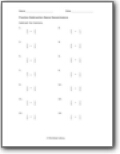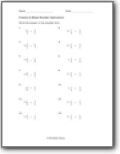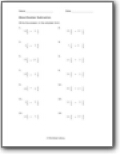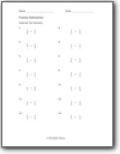# Fraction Subtraction Worksheets

#### How to Subtract Fractions

When you take a portion of a whole number, you are creating a fraction, any number of equal parts. So, what to do if you have to subtract two fractions? Well, it is simple, only when you know how to find out the LCM, the least common multiple. You need to make the denominators the same before you can subtract fractions. To do that, you need to take the Least Common Multiple of the denominators. Let's understand it better using an example. Example: 2/5 - 1/2. To subtract these, we need to look at the denominators, which are not the same. Find the common multiple of these two numbers. Here we know 10 is the LCM. You need to multiple the denominators of both fractions with a number that gives 10 as a result. Make sure to multiply the same number with the numerator.
(1×5)/(2×5) - (2×2)/(5×2)
5/10 - 4/10 = 1/10
This is how you subtract two fractions.###### Same Denominators

Where we can just focus on the numerator and get comfortable as we advance with this skill.###### Fraction & Mixed Number Subtraction

You will subtract a mixed number by a fraction. The denominators are all over the place.###### Mixed Number Subtraction

You will find the difference between two mixed numbers. A go way to approach these problems is to start by converting everything to a simple fraction.###### Unlike Fraction Subtraction

The denominators vary across these differences. They can be like or unlike.